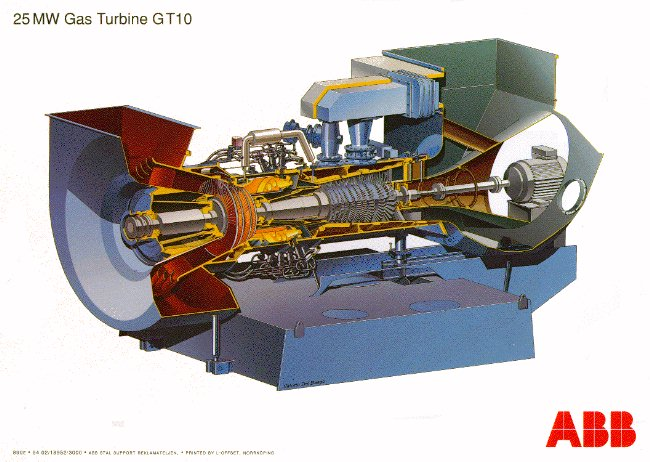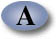UNIFIED ENGINEERING Fall 2004

Ian A. Waitz

Problem T7 (Unified Thermodynamics)

MIT’s gas turbine power plant operates on a Brayton cycle. The current operating conditions are shown at http://cogen.mit.edu/unified/. Assume the cycle is ideal and the gas behaves as an ideal gas with constant specific heats cp = 1.0035kJ/kg-K, and cv = 0.7165kJ/kg-K. The maximum temperature is 1400K and the minimum is 300K. The minimum pressure is 100kPa. The compressor pressure ratio is 13.

1. Calculate the pressure and temperature at each point of the cycle.
2. Calculate the ideal efficiency of the cycle.
3. How does the efficiency you calculated compare with the actual efficiency of the plant. [You can determine this from the data on the web page. If you get a full listing of variables, the fuel flow is listed under "total gas energy flow" and the net power is listed under "active load". If you use the buttons next to the picture, you need to add up the main and primary fuel flows to get the total gas energy flow. Note that 1 BTU is 1055 Joules, and 1 KBTU/s is 1000 BTU/s.] Why is the actual efficiency different from that you calculated in part b)?
4. A generalized schematic of a heat engine is shown at the end of Chapter 5. Sketch a similar representation for a refrigerator.
5. Could you use a Brayton cycle for a refrigerator? If no, why not? If yes, what would be required?